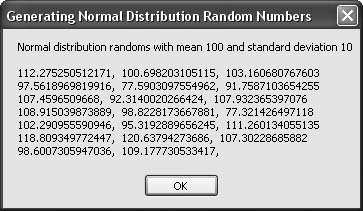# Recipe 6.29. Generating Normal-Distribution Random Numbers

#### Problem

You need to generate a sequence of pseudorandom numbers with a normal distribution, given the distribution's mean and standard deviation.

#### Solution

Sample code folder: Chapter 06\RepeatRandom

The BetterRandom class (see Recipe 6.26) sports a GetNextNormal() function. Two parameters passed to this function define the mean and standard deviation for the distribution of the generated values:

` GetNextNormal(mean, stdDev) `

#### Discussion

The following code creates a new instance of the BetterRandom object, which it then uses to generate 20 pseudorandom double-precision numbers with the desired normal distribution. As a programming exercise you might consider changing this code to display the mean and standard deviation for the returned values, to compare the results with the goal.

The generator object is created without passing a string to initialize the generator, so a unique sequence will be created every time this program is run:

` Dim result As New System.Text.StringBuilder Dim generator As New BetterRandom Dim mean As Double = 100 Dim stdDev As Double = 10 Dim counter As Integer result.Append("Normal distribution randoms with mean ") result.AppendLine(mean & " and standard deviation " & stdDev) result.AppendLine() For counter = 1 To 20    ' ----- Add one random number.    result.Append(generator.GetNextNormal(mean, stdDev))    If ((counter Mod 3) = 0) Then       ' ----- Group on distinct lines periodically.       result.AppendLine()    Else       result.Append(", ")    End If Next counter MsgBox(result.ToString()) `

Figure 6-29 shows the results of generating the 20 pseudorandom double-precision normal-distribution numbers.

##### Figure 6-29. Pseudorandom normally distributed numbers generated by the BetterRandom objectRecipe 6.26 shows the full code for the BetterRandom class.

There are many good references on the Web to learn more about random number generation (see, for example, http://random.mat.sbg.ac.at).Visual Basic 2005 Cookbook: Solutions for VB 2005 Programmers (Cookbooks (OReilly))
ISBN: 0596101775
EAN: 2147483647
Year: 2006
Pages: 400